Get our FREE VBA eBook of the 30 most useful Excel VBA macros.

Automate Excel so that you can save time and stop doing the jobs a trained monkey could do.

# Dynamic arrays and VBA user defined functions (UDFs)Office 365 subscribers now have Excel’s new calculation engine available to them, meaning lots of users suddenly have access to dynamic arrays.  This got me thinking about whether VBA user-defined functions (UDFs) can make use of the new functionality too.

I’m not an expert in this; I’m just sharing as much as I’ve learned to date.  If you’ve got a better or different approach, then please share it in the comments at the bottom.

If you’re not familiar with dynamic arrays, I recommend reading this post: Dynamic arrays in Excel.

I recommend you download the example file for this post.  Then you’ll be able to work along with examples and see the solution in action, plus the file will be useful for future reference.

Contents

## How do dynamic array calculations work?

To understand dynamic arrays, we first must understand how functions operate.  Each argument in a function accepts different data types (text, numbers, ranges, etc.).  If we put text where there should be a number, or a range where there should be text, we will encounter the #VALUE! error.

The data types required for each argument are visible in the Formula Arguments window.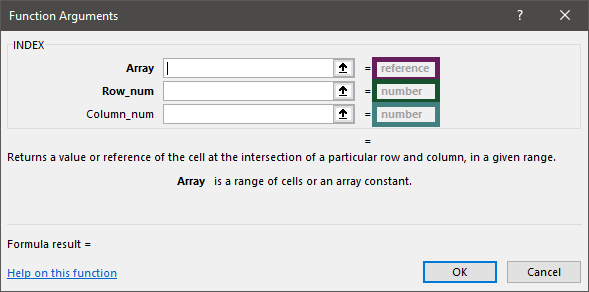The screenshot above shows the INDEX function has 3 arguments, each of which has a required data type:

• Array: Reference – can be a range or an array constant.
• Row_num and Column_num: Number – can be a cell containing a number or a single number constant.

Many functions, just like INDEX, contain arguments that expect a single value (this value is known as a scalar).  If we provide multiple values in that argument (such as range or array constant), Excel performs a calculation for every item in that array/range (this is known as lifting).  Excel has always operated in the way, but we only ever saw multiple results if we knew how to use Ctrl+Shift+Enter.  Instead, dynamic arrays display all the calculated results by spilling them into cells across and below.

Let’s look at two examples.

Example 1 – Non-dynamic array

The standard INDEX function calculates a single value, as shown by cell G4 below.  Notice that Row_num and Column_num arguments are individual values.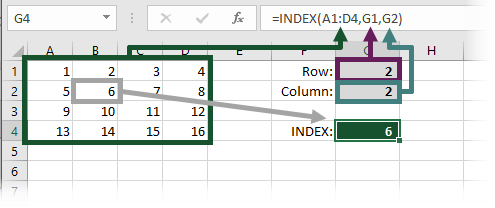Example 2 – Dynamic array

Now let’s give the Row_num and Column_num multiple values as ranges.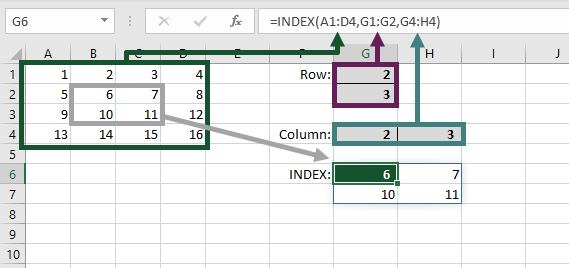Look at the screenshot above.  Cell G6 contains the formula, but since the Row_num and Column_num are both 2 values, the calculated results are pushed into the cells across and below.

## Using UDFs

Let’s try a user-defined function to see what happens.

The UDF used for the example calculates the volume of a sphere.  It only requires a single input, which is the radius.

```Function VolumeOfSphere(Radius As Double)

VolumeOfSphere = (4 / 3) * Application.WorksheetFunction.Pi() * Radius ^ 3

End Function```

When using a single value, the UDF operates correctly.  Look at the screenshot below, cell D2 calculates the volume using the radius in cell A2.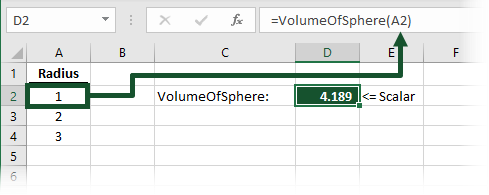Now let’s apply the dynamic array principles, and select multiple cells… oh dear, it doesn’t work.  The UDF has been given a range as an input (cells A2:A4), but it calculates as a #VALUE! error (cell D4).OK, now let’s see if we can make this work.## Create a dynamic array UDF

For a UDF to spill results into other cells, it needs to output an array.  Also, to accept multiple scalars in an argument, it needs to allow input arrays.  The size of the range/array is determined by the user through the arguments, therefore the easiest option for this is to use the Variant data type.

A reasonable understanding of VBA arrays is required to change the UDF into a dynamic array compatible function.  Check out my post about VBA arrays for further reading.

Here is the updated UDF.  As the Radius argument now allows multiple scalars, the function must be able to perform a calculation for each item in the range or array.

```Function VolumeOfSphereDA(Radius As Variant) As Variant

Dim i As Long
Dim j As Long
Dim test2DArray As Long
Dim tempArray() As Variant
Dim resultArray As Variant

'Resize Radius if 1D array
If TypeName(Radius) = "Variant()" Then
On Error Resume Next
test2DArray = UBound(Radius, 2)
On Error GoTo 0
If test2DArray = 0 Then
ReDim temp(1 To 1, LBound(Radius) To UBound(Radius))
For j = LBound(temp, 2) To UBound(temp, 2)
temp(1, j) = Radius(j)
Next j
End If
End If

'Force the resultArray to be the same size as the input array

'Calculate the result
For i = LBound(resultArray) To UBound(resultArray)
For j = LBound(resultArray, 2) To UBound(resultArray, 2)
resultArray(i, j) = (4 / 3) * Application.WorksheetFunction.Pi() _
* Radius(i, j) ^ 3
Next j
Next i

VolumeOfSphereDA = resultArray

End Function```

When multiple scalars are provided to the UDF above, the values spill into the cells across and below.  It behaves just like dynamic arrays should.  Look at the screenshot below, the formula in cell D6 accepts a range as input and spills the result into D7 and D8.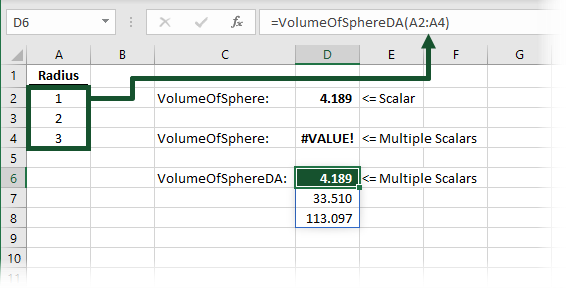The UDF will also works with array constants.

NOTES:

All ranges are automatically treated as having two-dimensions, even if they are just a single row.  By contrast, single row array constants, such as {1,2,3}, as always treated as having one-dimension.

As a result, the UDF converts one-dimension arrays to two-dimension arrays to prevent errors in the calculation process.

Thank you to two regular readers; Aryeh Nielsen and David Newell for helping me with this.

## Working with many multiple scalars

What happens when we have more than one argument which accepts scalars?  Native Excel uses a concept known as pairwise lifting.  This is where the rows and columns are matched by their relative position.  The first cells in each range are used to calculate the first result.  The second cells in each range are used to calculate the second result, etc, etc.

If one of the arguments contains a single scalar, Excel resizes the scalar to be an array with a consistent value for each element; this is known as broadcasting.  Once broadcasting has been completed the arrays are the same size, so pairwise lifting can continue as normal.

UDF’s don’t inherently use broadcasting, or pairwise lifting, we have to explicitly code in a way which creates the equivalent result.  This makes things significantly more complex.  To understand this, let’s change our UDF to calculate the volume of a cylinder.

The UDF takes two arguments both of which are scalars.

• Radius: The radius of the cylinder
• Length: The length of the cylinder
```Function VolumeOfCylinderDA(Radius As Variant, Length As Variant) As Variant

Dim i As Long
Dim j As Long
Dim test2DArray As Long
Dim temp As Variant
Dim resultArray As Variant
Dim resultArrayRows As Long
Dim resultArrayColumns As Long
Dim radiusArray As Variant
Dim lengthArray As Variant

'Resize Radius if 1D array
If TypeName(Radius) = "Variant()" Then
On Error Resume Next
test2DArray = UBound(Radius, 2)
On Error GoTo 0
If test2DArray = 0 Then
ReDim temp(1 To 1, LBound(Radius) To UBound(Radius))
For j = LBound(temp, 2) To UBound(temp, 2)
temp(1, j) = Radius(j)
Next j
End If
End If

'Resize Length if 1D array
If TypeName(Length) = "Variant()" Then
On Error Resume Next
test2DArray = UBound(Length, 2)
On Error GoTo 0
If test2DArray = 0 Then
ReDim temp(1 To 1, LBound(Length) To UBound(Length))
For j = LBound(temp, 2) To UBound(temp, 2)
temp(1, j) = Length(j)
Next j
Length = temp
End If
End If

'Convert ranges to arrays or retain if not range
lengthArray = Length

'Get dimensions of biggest array/range, ignore if not array/range
On Error Resume Next

If TypeName(radiusArray) = "Variant()" Then
If UBound(radiusArray) > resultArrayRows Then _
If UBound(radiusArray, 2) > resultArrayColumns Then _
resultArrayColumns = UBound(radiusArray, 2)
End If

If TypeName(lengthArray) = "Variant()" Then
If UBound(lengthArray) > resultArrayRows Then _
resultArrayRows = UBound(lengthArray)
If UBound(lengthArray, 2) > resultArrayColumns Then _
resultArrayColumns = UBound(lengthArray, 2)
End If

If resultArrayRows = 0 Then resultArrayRows = 1
If resultArrayColumns = 0 Then resultArrayColumns = 1

On Error GoTo 0

'Force the resultArray to be dimensions of biggest arrays
ReDim resultArray(1 To resultArrayRows, 1 To resultArrayColumns)

If TypeName(radiusArray) <> "Variant()" Then
ReDim temp(1 To resultArrayRows, 1 To resultArrayColumns)
For i = LBound(temp) To UBound(temp)
For j = LBound(temp, 2) To UBound(temp, 2)
temp(i, j) = radiusArray
Next j
Next i
End If

If TypeName(lengthArray) <> "Variant()" Then
ReDim temp(1 To resultArrayRows, 1 To resultArrayColumns)
For i = LBound(temp) To UBound(temp)
For j = LBound(temp, 2) To UBound(temp, 2)
temp(i, j) = lengthArray
Next j
Next i
lengthArray = temp
End If

'Calculate the result
For i = LBound(resultArray) To UBound(resultArray)
For j = LBound(resultArray, 2) To UBound(resultArray, 2)
resultArray(i, j) = Application.WorksheetFunction.Pi() _
* radiusArray(i, j) ^ 3 * lengthArray(i, j)
Next j
Next i

VolumeOfCylinderDA = resultArray

End Function```

The UDF has the following steps:

• One-dimension Radius or Length arrays are resized to two-dimension arrays.
• Radius and Length ranges are converted to arrays – this helps to simplify future calculations.
• Create an array to hold the result, the size is based on the maximum rows/columns of Radius and Length.
• Where Radius or Length is a single scalar, create a broadcasting effect by resizing to a two-dimension array.
• To calculate the result, the For Each loop matches values to create a pairwise lifting effect.

In the screenshot below, our UDF has applied logic that mimics pairwise lifting.  The first cell uses 1 and 10 as inputs, the second cell uses 2 and 20 as inputs, and so on.## Conclusion

Well, that’s all I have.  Hopefully, this will help you to turn your UDFs into dynamic array UDFs.

If you’ve got any further ideas about this I’d love to hear them.Get our FREE VBA eBook of the 30 most useful Excel VBA macros.

Automate Excel so that you can save time and stop doing the jobs a trained monkey could do.

Don’t forget:

If you’ve found this post useful, or if you have a better approach, then please leave a comment below.

Do you need help adapting this to your needs?

I’m guessing the examples in this post didn’t exactly meet your situation.  We all use Excel differently, so it’s impossible to write a post that will meet everybody’s needs.  By taking the time to understand the techniques and principles in this post (and elsewhere on this site) you should be able to adapt it to your needs.

But, if you’re still struggling you should:

1. Read other blogs, or watch YouTube videos on the same topic.  You will benefit much more by discovering your own solutions.
2. Ask the ‘Excel Ninja’ in your office.  It’s amazing what things other people know.
3. Ask a question in a forum like Mr Excel, or the Microsoft Answers Community.  Remember, the people on these forums are generally giving their time for free.  So take care to craft your question, make sure it’s clear and concise.  List all the things you’ve tried, and provide screenshots, code segments and example workbooks.
4. Use Excel Rescue, who are my consultancy partner.   They help by providing solutions to smaller Excel problems.

What next?
Don’t go yet, there is plenty more to learn on Excel Off The Grid.  Check out the latest posts:

## 5 thoughts on “Dynamic arrays and VBA user defined functions (UDFs)”

1.Aryeh Nielsen says:

There are four input possibilities, all of which must be converted to 2d Variant Arrays:
Column Range, Row Range, Constant Column Array {x;y;z}, Constant Row Array {x,y,z}

The below works for all forms of inputs. It determines which form the input is in, and converts them all to 2d Variant arrays. Perform at head of function, and use arrRadius instead of Radius elsewhere in function.

If TypeName(Radius) = “Range” Then ‘ If Range
If Radius.Rows.Count = 1 Then ‘ 1D Array as Row Range
Else
End If
ElseIf TypeName(Radius) = “Variant()” Then ‘ If Array

‘ Test for columns
On Error Resume Next
test = UBound(Radius, 2)
On Error GoTo 0

If test = 0 Then ‘ Row Array
Else ‘ Column Array
End If
End If

•Excel Off The Grid says:

Hi Aryeh,

Thank you for commenting, and point me in the right direction. Why do I find arrays in VBA so hard? Thanks for the code, I appreciate you taking the time to do this. I will review and add into the post.

•David N says:

I do something similar in many of my UDFs as well and for the same reasons.

2.David N says:

VBA treats single row arrays is being just one dimension, while single column arrays are seen as two dimensions. I think it has something to do with (or is at least similar to) how the Array function in VBA works.

For example…
Array(1,2,3) returns a one-dimensional array
Application.Transpose(Array(1,2,3)) returns a two-dimensional array

This is somewhat counterintuitive since a one row range and a one column range are both seen as two-dimensional in VBA. So in this case, your code is always expecting a 2-D array, and the #VALUE! error is because it cannot get the upper and lower bounds of the second dimension for a one row array constant.

•Excel Off The Grid says:

D’oh! Of course!!!

Thank you so much for your reply. In my mind I had a load of complex scenarios, none of which worked. It turned out to be something I should have known. Arrays catch me out once again…. won’t be the first, won’t be the last 🙂